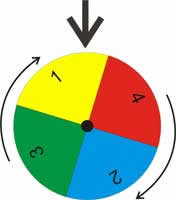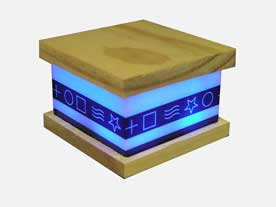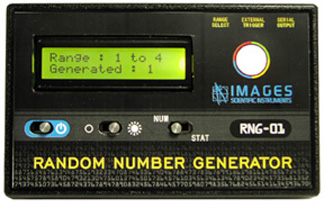Conscious Monkey™ Technologies

# How our Random Number Generators Work

How Our Random Number Generators Work

True random numbers are useful for data encryption (cryptography), statistical mechanics, probability, gaming, neural networks and disorder systems, to name a few applications.

Random numbers generated by a computer are notoriously random in an exact defined - patterns. So much so that they are referred to as pseudo-random numbers. The random numbers generated by this module are truly random.The way our RNG module operates is simple to understand. However, its fundamental function is best described using a mechanical analogy.

Imagine a sequence of numbers painted on the outer edge of a revolving wheel. There is a pointer on the outside of the wheel that points to the number at the top of the wheel. The wheel is set into motion, spinning very rapidly. Then at any given random moment in time the wheel is instantly stopped, and the number under the pointer becomes our random number. Once read, the wheel is set back into motion again for next random number generation.

The electronics follow pretty close to the mechanical analogy. The microcontroller spins the sequence of numbers internally. The random point in time when the sequence of numbers is instantly stopped is generated by a trigger. The trigger may be the detection of a radioactive particle by a Geiger Counter, or it could be that the thermal noise of a reversed bias diode just exceeded a pre-determined voltage threshold.**Background radiation by the way is an ideal quantum mechanical random time-delay generator because it is impossible to predict with any accuracy the exact moment a radioactive particle will be detected. Continuing with the Geiger counter trigger, when a particle is detected, a positive pulse is sent to Random Number Generator. The pulse is the trigger.

The trigger generates an interrupt in the microcontroller that stops the numbers from spinning. The number is read, displayed and sent out serially on the TTL port.Images employs random number generators in a number of products. The PSI Science lamps and the Stand Alone Random Number Generator RNG-01. The random number generators used in the PSI Science lamps are preset to output the numbers 1 through four. Where each number represents a particular color. This set-up makes it easy to use the PSI Science Lamp to test for PK and other PSI phenomena.

The Images Random Number Generator (RNG-01) can be set to determine how many numbers are painted on the wheel by setting the jumpers on the back of this module i.e. setting the range of random number being generated.There is a simple test to find the trueness of random numbers. If you add all the random numbers that are generated (let’s call this number N) and divide that by number of samples you added together (Let’s call this number S) the answer should be approximately 50% of your number range (Lets call this number R).

For example using a range of 1 — 10, so R = 10. The number of random number samples, is 100, so S = 100. We add those 100 random numbers together, that equals N. So N/S = .5 R In this case the number N when divided by S (100) should equal approximately 5. This would show an even distribution of random numbers, though out the range of 1 — 10.

Following link has a test program that will read a file of random numbers and analyze the randomness of the numbers in the file. http://www.fourmilab.ch/random/

**Background radiation consists of three sources; Cosmic radiation from the sun and stars. Terrestrial radiation from low levels of uranium, thorium, and their decay products in the soil, air and water. Internal radiation from radioactive potassium-40, carbon-14, lead-210, and other isotopes found inside our bodies.

Purchase a Random Number Generator